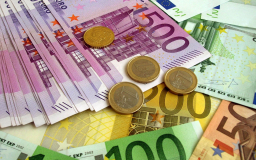# Daily balance

For the month of April, each checking account has a balance of $555 for 24 days,$2436 for one day, and \$243 for five days. What is the account's mean daily balance for April?

m =  565.7 USD

### Step-by-step explanation:Did you find an error or inaccuracy? Feel free to write us. Thank you!

Tips for related online calculators
Looking for help with calculating arithmetic mean?
Looking for a statistical calculator?
Need help calculating sum, simplifying, or multiplying fractions? Try our fraction calculator.

#### Grade of the word problem:

We encourage you to watch this tutorial video on this math problem: